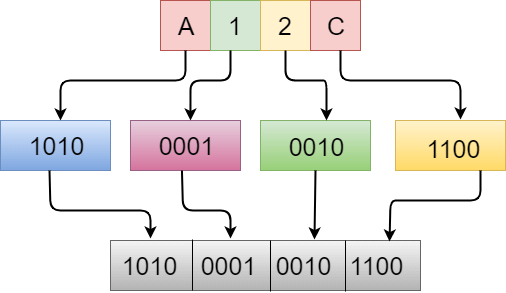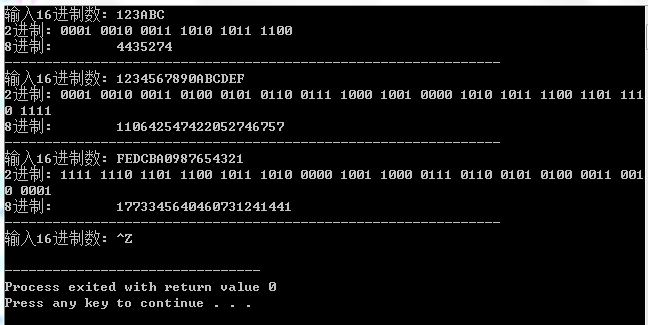• 十六进制转二进制,即拖即转,无需命令,超级好用.hex to bin
• labview十六进制转二进制，输入字符串控件是十六进制显示！不是正常显示那种超级简单的转换！labview
• 本文概述什么是十六进制十六进制是一种位置系统，以16为基数表示十六个不同的符号中的数字。这些不同的符号，即“ 0-9”代表从零到九的值，...十六进制数= A12C相当于A的二进制值是1010等于1的二进制值是0001等于...
本文概述什么是十六进制？十六进制是一种位置系统，以16为基数表示十六个不同的符号中的数字。这些不同的符号，即“ 0-9”代表从零到九的值，而“ A-F”代表从十到十五的值。什么是二进制？二进制数是一个以2为底的数字系统表示的数字，它使用两个符号，即0和1。例子假设十六进制数为A12C。现在我们计算十六进制数的二进制值。十六进制数= A12C相当于A的二进制值是1010等于1的二进制值是0001等于2的二进制值是0010相当于C的二进制值是1100因此，等效于A12C的二进制值为1010000100101100。注意：要将十六进制转换为二进制数，将对十六进制数的每个数字的二进制值进行求值并合并以获得给定十六进制数的二进制数。让我们通过一个例子来理解。#includevoid hextobin(char b[]);void main(){char hex[]="A12C";hextobin(hex);}void hextobin(char hex[]){int i=0;while(hex[i]){switch(hex[i]){case '0':printf("0000");break;case '1':printf("0001");break;case '2':printf("0010");break;case '3':printf("0011");break;case '4':printf("0100");break;case '5':printf("0101");break;case '6':printf("0110");break;case '7':printf("0111");break;case '8':printf("1000");break;case '9':printf("1000");break;case 'A':printf("1010");break;case 'a':printf("1010");break;case 'B':printf("1011");break;case 'b':printf("1011");break;case 'C':printf("1100");break;case 'c':printf("1100");break;case 'D':printf("1101");break;case 'd':printf("1101");break;case 'E':printf("1110");break;case 'e':printf("1110");break;case 'F':printf("1111");break;case 'f':printf("1111");break;}i++;}}在上面的代码中，我们要计算“ A12C”的二进制值。我们首先将此值存储在一个名为hex []的字符数组中，然后将该数组传递给hextobin()函数。 hextobin()将计算数字的二进制值，通过while循环迭代数组的每个元素，并通过switch语句查找每个对应元素的二进制值。输出量1010000100101100
展开全文• 本篇文章对c语言中十六进制转二进制显示的实现方法进行了详细的分析介绍，需要的朋友参考下
• Problem K: 十六进制转二进制 Description 二进制是计算机中数据的一种表示方法，只有两个符号0和1，分别代表十进制数值0和1。 十六进制是计算机中数据的一种表示方法，与十进制的对应关系是：十六进制的0～9对应十...
2017年山科C语言期末考试
Problem K: 十六进制转二进制
Description
二进制是计算机中数据的一种表示方法，只有两个符号0和1，分别代表十进制数值0和1。
十六进制是计算机中数据的一种表示方法，与十进制的对应关系是：十六进制的0～9对应十进制数值0-9，A～F对应十进制数值10-15。
现在你编写一个程序，完成一个十六进制数到二进制表示的转换。
Input
输入有多行，至EOF结束。每行一个十六进制整数n，n不超过100位十六进制数值。n只包括数字0～9和大写字母A～F。
Output
输出有多行，每一行输出与输入相对应，是n的二进制。
Sample Input
0123456789
ABCDEF

Sample Output
0000000100100011010001010110011110001001
101010111100110111101111

HINT
每1位十六进制值对应4位二进制值。
ans：
#include<stdio.h>
#include<string.h>

int main()
{
char s;

while(scanf("%s",s)!=EOF){
int num=0;
for(int i=0;i<strlen(s);i++){
int num=0;
if(s[i]>='0'&&s[i]<='9'){//判断是否为数字
num=s[i]-'0';
}else{//判断是否为字母
num=s[i]-'A'+10;
}
int a={0};//要转化为4位二进制数,用长为4的数组存放，先全赋值为0
int len=0;
while(num){//转化为4位二进制数
a[len++]=num%2;
num/=2;
}
printf("%d%d%d%d",a,a,a,a);、、倒着输出
}
printf("\n");
}
return 0;
}




展开全文• 存4位-取3位方法十六进制转二进制转八进制的思路最快的 最便于计算的 是 先转化成2进制，再转换成8进制： 0 0000 1 0001 2 0010 3 0011 4 0100 5 0101 6 0110 7 0111 8 1000 9 1001 A 1010 B 1011 C ...
存4位-取3位方法

十六进制转二进制转八进制的思路

最快的 最便于计算的
是 先转化成2进制，再转换成8进制：
0 0000
1 0001
2 0010
3 0011
4 0100
5 0101
6 0110
7 0111
8 1000
9 1001
A 1010
B 1011
C 1100
D 1101
E 1110
F 1111      搜索

先每四位一组转换成2进制
例如：
3BC24
分别对应到上表 就是：
3 0011
B 1011
C 1100
4 0100

连起来就是：
0011 1011 1100 0100

再按照每三个一组分组：
0 011 101 111 000 100

对应到8进制：
（其实跟上表的前一半是一样的）：
0 000    (0*4+0*2+0*1 = 0)
1 001    (0*4+0*2+1*1 = 1)
2 010    (0*4+1*2+0*1 = 2)
3 011    (0*4+1*2+1*1 = 3)
4 100    (1*4+0*2+0*1 = 4)
5 101    (1*4+0*2+1*1 = 5)
6 110    (1*4+1*2+0*1 = 6)
7 111    (1*4+1*2+1*1 = 7)

0 011 101 111 000 100
0__3__5__7__0__4
所以8进制就是35704

C语言程序的实现过程：

#include <stdio.h>
#include <string.h>
int eight, eight_n, two, two_n;
char sixteen;

int check()
{
int i;
for(i = 0; sixteen[i] != '\0' && sixteen[i] != '\n'; i++)
{
if(sixteen[i] < '0' || (sixteen[i] > '9' && sixteen[i] < 'A') || sixteen[i] > 'Z')
{
printf("请输入正确的16进制数: ");
return 0;
}
}
return 1;
}
int sixteen_two()
{
int i, two_num = 0;
for(i = 0; sixteen[i] != '\0' && sixteen[i] != '\n'; i++)
//转为2进制字符串放在two
{
switch(sixteen[i])
{
case '0': two[two_num++] = 0; two[two_num++] = 0;
two[two_num++] = 0; two[two_num++] = 0; break;
case '1': two[two_num++] = 0; two[two_num++] = 0;
two[two_num++] = 0; two[two_num++] = 1; break;
case '2': two[two_num++] = 0; two[two_num++] = 0;
two[two_num++] = 1; two[two_num++] = 0; break;
case '3': two[two_num++] = 0; two[two_num++] = 0;
two[two_num++] = 1; two[two_num++] = 1; break;
case '4': two[two_num++] = 0; two[two_num++] = 1;
two[two_num++] = 0; two[two_num++] = 0; break;
case '5': two[two_num++] = 0; two[two_num++] = 1;
two[two_num++] = 0; two[two_num++] = 1; break;
case '6': two[two_num++] = 0; two[two_num++] = 1;
two[two_num++] = 1; two[two_num++] = 0; break;
case '7': two[two_num++] = 0; two[two_num++] = 1;
two[two_num++] = 1; two[two_num++] = 1; break;
case '8': two[two_num++] = 1; two[two_num++] = 0;
two[two_num++] = 0; two[two_num++] = 0; break;
case '9': two[two_num++] = 1; two[two_num++] = 0;
two[two_num++] = 0; two[two_num++] = 1; break;
case 'A': two[two_num++] = 1; two[two_num++] = 0;
two[two_num++] = 1; two[two_num++] = 0; break;
case 'B': two[two_num++] = 1; two[two_num++] = 0;
two[two_num++] = 1; two[two_num++] = 1; break;
case 'C': two[two_num++] = 1; two[two_num++] = 1;
two[two_num++] = 0; two[two_num++] = 0; break;
case 'D': two[two_num++] = 1; two[two_num++] = 1;
two[two_num++] = 0; two[two_num++] = 1; break;
case 'E': two[two_num++] = 1; two[two_num++] = 1;
two[two_num++] = 1; two[two_num++] = 0; break;
case 'F': two[two_num++] = 1; two[two_num++] = 1;
two[two_num++] = 1; two[two_num++] = 1; break;
default: printf("输入错误信息\n");
}
}
return two_num;   //返回的是二进制个数
}
int two_eight(int two_num)
{//把转换后的8进制逆序存入数组
int i, eight_num = 0;
for(i = two_num; i >= 3; )
{
eight[eight_num++] = two[--i] + two[--i] * 2 + two[--i] * 4;
}
if(i == 2)
{
eight[eight_num++] = two[--i] + two[--i] * 2;
}
if(i == 1)
{
eight[eight_num++] = two[--i];
}
return eight_num;
}
void printf_two(int two_num)
{
int i;
printf("2进制: ");
for(i = 0; i < two_num; i++)   //输出2进制数列
{
printf("%d", two[i]);
if((i+1) % 4 == 0)
printf(" ");
}
printf("\n");
}
void printf_eight(int eight_num)
{//得到八进制的个数
eight_num--;
printf("8进制:        ");
while(eight[eight_num] == 0)
eight_num--;
for(; eight_num >= 0; eight_num--)
{
printf("%d", eight[eight_num]);
}
printf("\n-----------------------------------------------------------------\n");
}
int main()
{
printf("输入16进制数: ");
while(gets(sixteen))
{
two_n = 0;
eight_n = 0;
//检验输入的十六进制数是否符合规定
if(check() == 0)
continue;

two_n = sixteen_two(); //读取十六进制，算出二进制存在two数组和个数two_n

//读取二进制个数，算出八进制存在eight数组和返回个数eight_n
eight_n = two_eight(two_n);
printf_two(two_n);     //输出二进制
printf_eight(eight_n); //输出八进制
printf("输入16进制数: ");
}
return 0;
}展开全文c语言
• 从一开始就经常提到“一个十六进制字节”，然而一开始就是迷惑的，直到现在。 一个十六进制字节，比如：FF。周围的人经常说这是一个十六进制字节。然后我就想，这不是两个字符吗，分别是F 和F，一个字符是一个字节...
做项目也将近一年的时间了。从一开始就经常提到“一个十六进制字节”，然而一开始就是迷惑的，直到现在。
一个十六进制字节，比如：FF。周围的人经常说这是一个十六进制字节。然后我就想，这不是两个字符吗，分别是F 和F，一个字符是一个字节，两个字符是两个字节，怎么就成一个十六进制字节，就成一个字节了。就是这种想法，整整困扰了我将近一年的时间。
这几天在写论文，要在数据压缩上找点可写之处，分析数据的组成和格式。结果焦点还是在一个十六进制字节。使用半字节压缩法，很适合项目和论文的需要。而且项目当中就是这样做的。
经查，一个十六进制字节是这样定义的：char a=0xFF=255.
又查，一个字节总是由一对十六进制数来表示，上面的a是一个字符，即一个字节；十六进制数F和F组成以一对十六进制数。这对十六进制数转换为二进制数为：11111111，正好是8 bits，一个字节。

讲讲二进制、字节、16进制
1.二进制就是逢二进一，只有0和1。 一个字节就是一个英文字母、阿拉伯数字或半个汉字所占的空间（一个汉字占2个字节）。
16进制就是逢16进一，只有0123456789abcdef这16个数字（或子母）。
由于电脑里都是二进制的数，而16进制与二进制有简单的转换关系，所以很多书上都写16进制的数。
一个字节由8个二进制组成，它所能表示最大十六进制为： ?FF(16进制)=256(10进制)=11111111(2进制)。8位二进制最大为255,转换为16进制则为ff。

2.字节是电脑里用于存储的最小单位..?

一个字节占用八位的二进制数，及一个字节等于8位二进制。所以..二进制是计算机的一种语言.字节是一个单位.所以说：计算机里面存储数据是以字节为单位的，即8个二进制代码。而不是以二进制代码储存的。
由于二进制数在使用中位数太长,不容易记忆,所以又提出了十六进制数.
3.在最早的时候，是约定的8位二进制为一个字节，和ASCⅡ码无关，可以表示256以内的数。

在
机器内部，8位或者16位对它来说都一样，都是1或0，而程序设计的时候，人为的要求机器按8位或16位来处理。16位的音乐文件所包含的信息比8位的多
得多（差256倍），因此你也可以将16位二进制数看作音乐文件的一个单元，但约定成2个字节。就相当于统一了单位，便于交流。

4.十六进制转换二进制，举例：
?问：十六进制(A5)转换成二进制？
??解答：
?方法一、A*16的1次方+5*16的0次方得出十进制。然后拿十进制再转二进制，这样的话 比较麻烦。?

?方法二、16进制中A用二进制表是多少？A代表10 ，就是二进制的 1010。 我例个表出来。十六进制以 4 位为一小段.8进制以 3 位为一小段 。0 0000 1 0001 2 0010 3 0011 5 0101 ... 9
1001 A 1010 B 1011 C 1100 ... F 1111 所以A5就是 1010
0101。
对方法二，进行解释：
十六进制中的 F 转换成二进制 就是 1111 所以把十六进制中每个位数上的数单独转换成一个4位的二进制数，再做拼接 A5 A
--1010 5 --0101 所以 A5 －1010 0101。

转载于:https://www.cnblogs.com/Alight/p/4088715.html
展开全文• /* 六位数十六进制转二进制 */ #include <iostream> using namespace std; int main() { char a; //定义的是数组长度，最后一个数组元素为a int i; for(i = 0; i < 6; i++) { cin...
• c++ 十六进制转二进制数I recently introduced the Decimal Number System, the one we are used as humans. 我最近介绍了小数系统 ，即我们用作人类的系统。 As I said in that post, as humans we commonly ...python java 数据库 算法 人工智能
• 进制转二进制 十进制整数转二进制 十进制整数转换成二进制采用“除2倒取余”，十进制小数转换成二进制小数采用“乘2取整”。 例题： 135D = __ B 解析：如下图所示，将135除以2，得余数，直到不能整除，然后再...计算机基础
• 十六进制转二进制我们可以先把十六进制转化成我们熟悉的十进制，然后在由十进制转成我们所熟悉的二进制。这样写好处是，代码简洁易懂易读。 以下是本人所写的代码： #include&lt;stdio.h&gt; #include&...
• 十进制转二进制，十六进制转二进制的方法虽然可以直接借助一些在线转化工具，但是老是会混淆里面的具体含义，搞的我脑子都乱了 比如43转化成二进制，先要看43是什么数，要是43是个十进制数，那很简单，就是0010 ...
• 感谢百篇之中翻到这篇！ 为了感谢你们，特意给大家一个福利------------- 记住口诀：三四五六七 什么意思呢 0 的ascii码值是 30H ...十六进制转二进制具体代码 DATA SEGMENT  DATA_0X DW 0F804H ...
• 可以实现十六进制转换成二进制，使用java写的，是可执行的jar包
• 第四章第十二题（十六进制转二进制）(Hex to binary) 4.12（十六进制转二进制）编写一个程序，提示用户输入一个十六进制数，显示其对应的二进制数。对于不正确的输入数字，提示非法输入。 下面是一个运行示例： ...java
• 一个简洁易用好用简单的十六进制转二进制，hex2binary hex to binary hex2bin hex2binary。用法很简单，把你要转到hex文件 文档 拖到这个程序的图标上就会在当前目录下生成对应名字的bin文件（二进制文档）。自持hex...
• 基础练习 十六进制转八进制 时间限制：1.0s 内存限制：512.0MB 问题描述 　给定n个十六进制正整数，输出它们对应的八进制数。 输入格式 　输入的第一行为一个正整数n （1&lt;=n&lt;=10）。 　接下来n行...
• 　接下来n行，每行一个由0~9、大写字母A~F组成的字符串，表示要转换的十六进制正整数，每个十六进制数长度不超过100000。 输出格式 　输出n行，每行为输入对应的八进制正整数。 注意 　输入的十六进制数不会有前导0...算法基础
• 是在做一个Android应用用到了这些代码，我知道java有针对整数的各种进制转化，但我要的是浮点数。请大神们帮帮忙嘞。。java android
• 将一个十六进制数转化为一个二进制数。 输入描述 Input Description 一行，一个十六进制下的整数 输出描述 Output Description 这个十六进制数对应的二进制结果 样例输入 Sample Input FEDCBA 样例输出 ...
• 十六进制数（FB93）用二进制输出，汇编的一个题目
• 二进制十六进制十进制转换 将十六进制数转换为十进制数，并将十进制数转换为十六进制数 这是一个非常简单的脚本，可将十进制数字转换为十六进制值，并将十六进制值转换为十进制数字。 此示例后面的示例演示了如何将...字符串 javascript java python js...